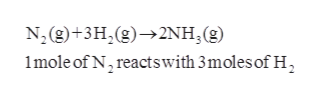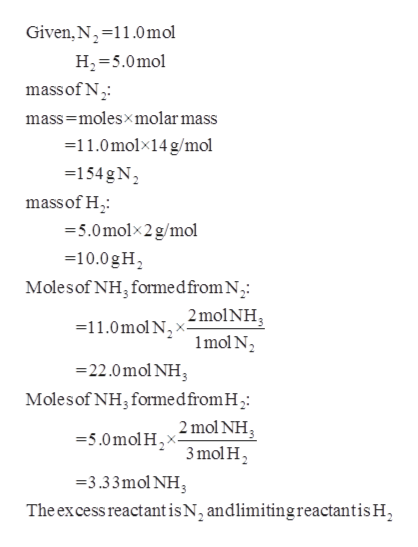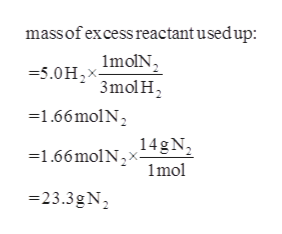Nitrogen N2 gas and hydrogen H2 gas react to form ammonia NH3gas. Suppose you have11.0molofN2and5.0molofH2in a reactor. Suppose as much as possible of theN2 reacts. How much will be left? Round your answer to the nearest 0.1 mol.

Question

Nitrogen

N2

gas

and hydrogen

H2

gas react to form ammonia

NH3

gas. Suppose you have

11.0
mol

of

N2

and

5.0
mol

of

H2

in a reactor.

Suppose as much as possible of the

N2

reacts. How much will be left? Round your answer to the nearest

0.1

mol

.

Step 1

The balanced equation for the reaction of formation of ammonia can be given ashelp_outlineImage TranscriptioncloseN, (g)+3H,(g)2NH, (g) 1mole of N, reactswith 3moles of H fullscreen
Step 2

From the given moles of reactants, mass is calculated and with each reactant the number of moles of ammonia formed can be calculated.help_outlineImage TranscriptioncloseGiven,N 11.0mol H2 5.0mo massof N mass-molesxmolar mass = 11.0molx14 g/mol =154 gN massof H -5.0molx2g/mol =10.0gH Molesof NH, formedfrom N =11.0mol N x2molNH, mol N 22.0molNH Molesof NH, formedfromH2 5.0molHmol NH3 3 mol H 2 3.33mol NH The excess reactant is N, andlimitingreactantis H fullscreen
Step 3

The mass of excess reactant that is used up ...help_outlineImage Transcriptionclosemassof excess reactant usedup: 5.0HImoIN, -50H2 3molH -1.66molN 1.66molN14gN 1mol -23.3gN fullscreen

Want to see the full answer?

See Solution

Want to see this answer and more?

Our solutions are written by experts, many with advanced degrees, and available 24/7

See Solution
Tagged in

Chemistry